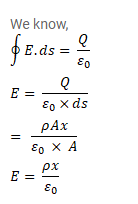# A nonconducting sheet of large surface area and thicknessQuestion:

A nonconducting sheet of large surface area and thickness $d$ contains uniform charge distribution of density p. Find the electric field at a point $\mathrm{P}$ inside the plate, at a distance ${ }^{x}$ from the central plane. Draw a qualitative graph of $E$ against $x$ for $0<$ $\mathrm{x}<\mathrm{d}$.

Solution: